JOURNAL OFSYNCHROTRONRADIATION
ISSN: 1600-5775
Volume 16| Part 2| March 2009| Pages 152-162

## Absorbed dose calculations for macromolecular crystals: improvements to RADDOSE

aLaboratory of Molecular Biophysics, Department of Biochemistry, University of Oxford, South Parks Road, Oxford OX1 3QU, UK, and bSwiss Light Source, Paul Scherrer Institut, CH-5232 Villigen PSI, Switzerland
*Correspondence e-mail: elspeth.garman@bioch.ox.ac.uk

(Received 29 August 2008; accepted 27 November 2008)

Radiation damage is an unwelcome and unavoidable aspect of macromolecular crystallography. In order to quantify the extent of X-ray-induced changes, knowledge of the dose (absorbed energy per unit mass) is necessary since it is the obvious metric against which to plot variables such as diffraction intensity loss and B factors. Significant improvements to the program RADDOSE for accurately calculating the dose absorbed by macromolecular crystals are presented here. Specifically, the probability of energy loss through the escape of fluorescent photons from de-excitation of an atom following photoelectric absorption is now included. For lighter elements, both the probability of fluorescence and of its subsequent escape from the crystal are negligible, but for heavier atoms the chance of fluorescence becomes significant (e.g. 30% as opposed to Auger electron decay from a K-shell excited iron atom), and this has the effect of reducing the absorbed dose. The effects of this phenomenon on dose calculations are presented for examples of crystals of an iron-containing protein, 2-selenomethionine proteins, a uranium derivatised protein, and for a nucleic acid sample. For instance, the inclusion of fluorescent escape results in up to a 27% decrease in the calculated absorbed dose for a typical selenomethionine protein crystal irradiated at the selenium K-edge.

### 1. Introduction

X-ray diffraction is an important tool for the structure determination of biological macromolecules. For a full understanding of the chemistry of macromolecular function, it is essential to determine the structure with minimum damage to the sample. The ionizing effects of X-rays have been a major concern in macromolecular crystallography (MX) for many years (Blake & Phillips, 1962). Although cryo-cooling (Low et al., 1966; Rodgers, 1997; Garman & Schneider, 1997) slows the damage rate compared with at room temperature, radiation damage at 100 K is a limiting factor in some structure determinations. Since the late 1990s the need to understand and quantify radiation damage has gained increasing importance with the widespread use of high-brilliance MX synchrotron beamlines (Ravelli & Garman, 2006). The various symptoms of radiation damage include a decrease in diffraction intensity, a loss of resolution, increasing B factors, expansion of the unit-cell volume, discolouration of the crystal and specific structural damage.

MX diffraction experiments that rely on the exploitation of anomalous scattering for phase determination, such as MAD (multi-wavelength anomalous dispersion) and SAD (single-wavelength anomalous dispersion) phasing, are very sensitive to radiation damage, since the non-isomorphism induced both by unit-cell expansion and damage to specific amino acids by the X-ray beam causes particular problems (González, 2007). Even before the intensity decay of the crystal is observable, specific structural damage is induced, starting with the breakage of disulphide bridges, followed by decarboxylation of aspartates, glutamates and the C-terminus, and loss of the hydroxyl group from tyrosines (Ravelli & McSweeney, 2000; Weik et al., 2000; Burmeister, 2000). In spite of these observations, radiation damage can also be a benefit in biological investigations (Dubnovitsky et al., 2005; Ravelli & Garman, 2006). Phase determination can be performed by using specific radiation damage (Ravelli et al., 2003), and more recently dynamic information relating to the functioning of a protein has been demonstrated on acetylcholinesterase, through observation of radiation damage at 100 K and 150 K (Colletier et al., 2008).

Dose is defined as the energy absorbed per unit mass by, in this case, a crystal. Most X-ray photons that interact with the crystal deposit energy into it causing radiation damage. For instance, for a 100 µm-thick protein crystal containing no heavy elements and bombarded with 12.4 keV X-rays, 2% of the beam will interact, of which only 8% will be elastically scattered (diffraction) with the rest losing some or all of their energy in the sample [84% by the photoelectric effect and 8% by inelastic (Compton) scattering]. At higher X-ray energies the absorption decreases and a lower dose is deposited in the crystal, but the diffraction intensity is concomitantly reduced.

The dose that can be tolerated by a macromolecular crystal before it loses half of its diffraction intensity was predicted by Henderson (1990) to be 20 MGy, a value derived from observations of radiation damage by 100 keV electrons to biological samples in electron microscopy. Owen et al. (2006) measured an experimental upper dose limit for MX of 30 MGy, corresponding to a reduction of the average diffraction intensity to 0.7 of its original value, and after which the biological information inferred is likely to be compromised. The absorbed dose describes the physics of the energy loss but does not include consideration of the chemistry of the radiation effects that occur in the crystal. For instance, the presence of particularly susceptible residues at crystal contacts may result in the lattice collapsing (Murray et al., 2005) well before the dose limit of 30 MGy is reached. Thus a crystal may be able to tolerate the dose limit, but it is very unlikely to survive beyond it.

It is generally accepted that the dose at which a data collection should be stopped is primarily defined by the point where biological information is lost owing to the poor quality of data. The resulting electron density maps can be used both to qualitatively and quantitatively assess the damage to the structure.

The program RADDOSE (Murray et al., 2004) was written to calculate the absorption cross sections for macromolecular crystals at differing X-ray energies. From the beam parameters, chemical composition and physical dimensions of the crystal, the dose can be computed and can be used in both estimating the length of time available for data collection at a particular beamline and for deriving a data collection strategy that will result in the maximum data for the lowest dose (and thus minimizing the overall radiation damage).

### 2. Computing the dose: RADDOSE

The purpose of this report is to describe some changes, improvements and additions made to the program RADDOSE. Details of the basic methodology are given in the original RADDOSE paper (Murray et al., 2004), but a brief description is given here for completeness. Given the physical and chemical composition of the crystal, beam characteristics and data collection parameters, the program estimates the absorbed dose (J kg−1) and hence the time taken to reach the Henderson limit (20 MGy; Henderson, 1990) or any other dose limit specified by the user.

The photons in the incident X-ray beam are both attenuated and absorbed in the crystal. The energy absorbed is a function of the incident energy, its flux and of μabs, the X-ray absorption coefficient of the crystal, which in turn is crucially dependent in the constituents of the crystal. The interaction of incident X-ray photons at energies commonly used in MX (5–18 keV) can be classified into three types: elastic (coherent, Thomson), inelastic (incoherent) and that arising from the photoelectric effect. Photons that are inelastically (Compton) scattered contribute little to absorption (and are neglected in RADDOSE) leading to a small underestimate of the absorbed dose. The prominent event at energies of interest to macromolecular crystallographers is the photoelectric effect, as the entire energy of the photon may be deposited in the crystal, leading to radiation damage.

Whereas the total cross section, σ (consisting of photoelectric, elastic and inelastic parts), is used to obtain the attenuation coefficient, only the photoelectric cross section is used to compute the absorption coefficient. The total linear attenuation coefficient of a crystal, μatt, can be calculated from the sum of the atomic cross sections for all the different atom types (j = 1 to n) in the unit-cell volume V,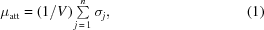where σ = σThomson + σCompton + σphotoelectric.

This parameterization assumes that the cross section of an atom is independent of its environment. While this is valid for light atoms, for heavy atoms close to absorption edge energies this results in a less accurate estimation of the true experimental cross section. A better value in such cases can be obtained by collecting a fluorescence scan over the absorption edge of the heavier element prior to the diffraction experiment, to obtain the true shape of the edge. The magnitude of the fluorescence is directly proportional to the photoelectric absorption coefficient. Therefore, accurate values of σphotoelectric can be estimated from the X-ray fluorescence spectrum, which is scaled to the theoretically tabulated values of the absorption coefficients far from the absorption edge.

The dose calculation is performed in three stages. First, from the input information, the program calculates the number of atoms of each element present in the unit cell assuming five C atoms, 1.35 N atoms, 1.5 O atoms and 8 H atoms for the composition of an amino acid; 9.75 C, 4 N, 6 O, 11.75 H and 1 P atom for a DNA nucleotide; and 9.5 C, 3.75 N, 7 O, 11.25 H and 1 P for an RNA nucleotide. The constituents of the solvent may also be specified (in mM or in numbers of atoms) so that the precise composition of material in the crystal channels can be calculated. If no solvent information is provided in the input, the non-protein volume of the unit cell is filled with water. Next, the photoelectric cross sections of the different elements present are used to calculate the absorption coefficient of the crystal from a library of photoelectric cross sections for all atoms in the periodic table (McMaster et al., 1969) which are held in the subroutine mucal.f (Badyopadhyay, 1995). When appropriate, RADDOSE can correct the absorption coefficients of anomalously scattering atoms by normalizing them to the f ′′ values calculated by the program CHOOCH (Evans & Pettifer, 2001) following an experimental fluorescence scan.

The attenuation coefficient is computed by summing up the contributions from photoelectric, Compton and elastic scattering. Using the photon flux and energy, beam shape and size, exposure time per image and the number of images, the total number of photons incident on the crystal of specified size is calculated. The default beam profile is a top-hat function, but if the intensity profile of the X-ray beam is not uniform an elliptical Gaussian function can be modelled with the knowledge of the FWHM of the beam profile in two orthogonal directions. The calculation assumes that the crystal is stationary throughout the data collection; this problem will be revisited (see §4).

The calculated absorption coefficient and number of photons incident on the crystal are then used to compute the number of photons absorbed by the crystal and, subsequently, the amount of energy deposited in the crystal. The temperature rise induced by the X-ray beam in the sample is also calculated, using the simple isothermal lumped model' (Kuzay et al., 2001).

In the final step, the absorbed dose (energy deposited divided by the mass of the exposed part of the crystal) is computed.

The dose can be expressed as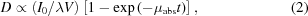where D is the dose absorbed (energy per unit mass) by a crystal of thickness t, irradiated volume V and absorption coefficient μabs for an incident beam of wavelength λ and initial intensity I0.

As an aid to a quantitative understanding of the relationship between diffraction and energy loss, Arndt (1984) proposed a diffraction efficiency measure, IE, based on the diffracted intensity, Iscatt, divided by the energy deposited (Iscatt/Eabs) as shown in equation (3),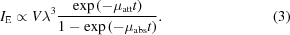Murray et al. (2004) further suggested using the diffracted intensity per absorbed dose, known as the diffraction-dose efficiency, IDE (= Iscatt/D),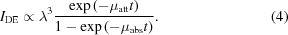This quantity is output by RADDOSE and can be useful for optimizing the experimental parameters, for instance giving an indication of the change of signal per dose as a function of energy.

The current version of RADDOSE is widely used to calculate absorbed dose to quantify the effects of radiation damage in structural biology (Pearson et al., 2007; Fioravanti et al., 2007; Banumathi et al., 2004). Increasingly, it is also being implemented to assist in the design of optimal complete data collection strategies [e.g. BEST (Bourenkov & Popov, 2006), Web-Ice (Gonźalez et al., 2008)].

RADDOSE has now been updated to compute the probability that fluorescent photons from the decay of atoms excited by the photoelectric effect may escape, thus obtaining a better estimate of the absorbed dose. This is described in §4In addition, to enhance the ease of use and the uptake of RADDOSE in non-MX fields, the CCP4 library routines that perform basic input and output operations have been replaced by local subroutines. The time taken to reach the Henderson dose limit of 20 MGy (Henderson, 1990) is calculated as previously, but the experimental dose limit of 30 MGy (Owen et al., 2006) is now also computed.

### 3. Accounting for X-ray fluorescence escape

Previous versions of RADDOSE assume that, if a photoelectric electron is produced in the crystal, all the energy of the X-ray causing this event is deposited within the crystal. In fact, following ejection of a photoelectron, an atom is left in a short-lived excited state which can either decay by emission of an Auger electron or by emitting a fluorescent X-ray (Fig. 1). If the excited state decays via the fluorescence pathway, the X-ray produced has a finite probability of escaping from the crystal (depending on its size and constituents, and on the energy of the fluorescent X-ray). Thus not all the energy of the incident X-ray is deposited in the crystal, and the absorbed dose calculated by RADDOSE is an overestimate.Figure 1 The phenomenon of X-ray fluorescence. The X-ray photon interacts with (a) a K-shell (n = 1) or L-shell (n = 2) electron in an atom (b) via the photoelectric effect, ejecting an electron, and the atom can relax either (c) via the Auger effect (top) by ejection of an electron or by X-ray fluorescence (bottom). The lifetime of K holes can be estimated from Auger line-widths and are of the order of femtoseconds (Krause & Oliver, 1979).

Fluorescent X-rays can only be produced if the incident X-ray energy is greater than any of the absorption-edge energies (K, L or M) of the atoms present so that the atom can undergo excitation by the photoelectric effect. All the elements commonly present in macromolecular crystals, except atoms with Z > 62, have LIII-edge energies at less than 6 keV and can therefore undergo LIII-photoelectric excitation (see Fig. 2). The relative probabilities of K- and L-shell excitation for each atom are known and tabulated (Table 1) (Krause, 1979).

 Table 1Summary of the different probabilities and yields for K-shell X-ray fluorescence for elements routinely of interest in MX
 For elements heavier than (but not including) cadmium, the equivalent values for the LIII-edge are provided.
Element Atomic number Z Edge ratio (r) K- or LIII-shell edge energy (keV) Probability of K- or LIII-shell ionization (A) Probability of K- or LIII-shell fluorescence yield (B)
Si 14 10.3 1.84 0.9 0.05
S 16 9.63 2.47 0.9 0.078
Fe 26 7.9 7.11 0.9 0.34
Cd 48 6.28 26.7 0.84 0.84
I 53 2.95 4.56 0.66 0.08
Xe 54 2.92 4.78 0.66 0.085
Gd 64 2.75 7.24 0.64 0.155
Ta 73 2.61 9.88 0.62 0.243
Pt 78 2.56 11.56 0.6 0.3
Hg 80 2.54 12.3 0.61 0.33
U 92 2.4 17.17 0.58 0.5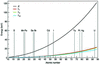Figure 2 K (black line), LI (red line), LII (green line) and LIII (blue line) edge energies for elements with atomic numbers Z = 11 to Z = 94.

As described above, once excited via the photoelectric effect an atom can decay either by the Auger process or by X-ray fluorescence. The probability for the latter process is negligible for light elements, but increases with Z, the atomic number, as shown in Fig. 3, becoming significant (>10%) for chlorine (Z  =  17) and rising to 58% for selenium and 97% for uranium. A careful inspection of the probabilities reveals that for elements with Z < 36 (krypton) the probability of LIII-shell fluorescence yield is very low (less than 2%) and can therefore be neglected.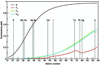Figure 3 Probability of K (black line), LI (red line), LII (green line) and LIII (blue line) shell fluorescence yield as opposed to Auger electron decay as a function of atomic number.

The fluorescent X-rays produced in this process will have a finite probability of escaping from the sample, depending on their energy and the crystal thickness. Thus energy will be lost from the crystal and the absorbed dose will be lower than if this effect were not taken into account.

If all the various atom types in the crystal have K-edge energies that are lower than the incident X-ray energy, then K-shell fluorescence excitation cannot occur and all the energy of the photon absorbed in the photoelectric effect is deposited in the crystal. In MX, especially when phasing using the MAD technique, data are usually collected at energies on, or above, the edge energies of a specific element. Thus, a proper estimate of the X-ray fluorescence escape is necessary for arriving at accurate values of absorbed dose.

The wavelength dependence of the photoelectric cross section of atoms is well known in MX as the imaginary part of the anomalous scattering factor (f ′′) and is directly proportional to the photoelectric cross section. Given a specific element, the energy that maximizes f ′′, and hence gives maximum anomalous signal, also maximizes the absorption of the incident photons, consequently reducing the lifetime of the crystal compared with values obtained at incident energies below the absorption edge of the specific element. There are two contributions to the photoelectric cross section, μpe, of an atom: the K-shell cross section (μK) and the L-shell cross section (μL). For very heavy elements the M-shell may also have to be considered, but it can be seen from Fig. 3that the probabilities of fluorescence, which are below the L-shell values, will be negligible. When taken together with the probability of M-shell excitation, which again will be below that for the L-edge shown in Fig. 2, it is clear that the M-shell contribution can be neglected. Additionally, if produced, the M-shell fluorescent X-ray energy is so low that the photon is very likely to be absorbed in the crystal rather than escape from it. M-shell values are not shown in Figs. 2and 3since the data are not available.

At energies below the K-edge, μK = 0 and all the photoelectric interactions are via the L-shell with a significantly lower yield. Above the K-edge, the relative contribution of μK and μL to μpe can be calculated by evaluating the increase in the photoelectric cross section at the K-edge and extrapolating this to higher energies (Reed, 1993).

The fraction of μpe attributable to K-shell ionization (above the K-edge) at an incident X-ray energy E is equal to (see Fig. 4)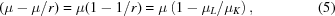where r is the edge ratio', defined as μK/μL, and μK and μL are the photoelectric cross sections for the K- and L-shell, respectively, at the K-edge. The fraction μK/μL was obtained using the data from the cross-section database for X-rays (Berger et al., 2005). In the parameterization implemented in RADDOSE, the fraction of the total ionization which is due to K-shell (or L-shell) excitation is defined as the probability A, and is constant with incident energy for each element. For heavier elements, starting with iodine (Z = 53), the edge ratio for the LIII-edge is obtained by calculating the ratio of photoelectric absorption at the LIII-edge to that of the MI-edge. The edge ratios for LI and LII are calculated in a similar way. The values of edge ratios, and K- and L-edge energies for some elements of interest, are listed in Table 1.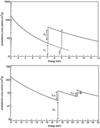Figure 4 The photoelectric cross section of iron (top) and uranium (bottom) as a function of energy. μK, μL and μM are the contributions of the K-, L- and M-shell cross sections to the total photoelectric cross section, and r is the edge ratio = μK/μL or μL/μM in the cases of iron and uranium, respectively.

As described above, after K-shell ionization an atom relaxes by allowing an L-shell electron to fill the K-shell vacancy. The excess energy remaining after this process can be emitted from the atom via another L-shell electron (Auger) or by a photon (X-ray fluorescence). The K-shell (or LIII-shell) fluorescence yield of an atom (obtained from the subroutine mucal.f) gives the relative probabilities of these two processes and is defined as probability B (see Fig. 3).

Depending on the thickness of the crystal, there is then a possibility that the fluorescent X-ray escapes from the sample; for crystals of a few micrometres in size, a significant proportion could do so. In fact, for such small crystals the original photoelectron might even be able to leave the crystal, and provide significant reduction in radiation damage (Nave & Hill, 2005; Cowan & Nave, 2008). Counter-intuitively, at a given incident X-ray energy a larger crystal suffers a smaller absorbed dose than a smaller one, as a result of the attenuation of the beam by the crystal which gives a lower average energy loss per kilogram of sample (i.e. lower dose). This is especially important at lower energies (<7 keV), at which the absorption can be high, although these energies are not commonly used in MX.

In order to calculate the probability of fluorescent X-ray escape (referred to as probability C), some assumptions must be made regarding the path length to be travelled, and thus on the site of production of the fluorescent X-ray. For the purpose of the RADDOSE calculation, the fluorescent photons are all assumed to have been emitted halfway through the depth of the crystal. As X-rays will, in reality, interact throughout the crystal depth, this central emission hypothesis is an approximation. A full treatment of this problem would require a volume integral over the probability of fluorescent escape for each position (a function of the distance travelled by the beam through the crystal, x), and a spherical polar coordinate integral over the angle of emission throughout the whole crystal. For this integral to be correctly calculated the dimensions and orientation of the crystal would have to be accurately known. The central emission hypothesis is, however, not unreasonable since fluorescent X-rays are believed to be isotropically emitted from excited atoms. Thus, for an atom near the edge of the crystal, fluorescent X-rays will either have a much greater chance of escape than those emanating from the centre, or a much lower chance depending on the direction of emission. Therefore, the approximation that all fluorescence X-rays are produced halfway through the crystal does not introduce a significant error into the dose calculation, and implementation of an integration of the escape probability over the volume was thought to be un­necessary and beyond the scope of this study.

Since the tracks of the fluorescent X-ray photon are linear in the crystal (unlike those of the photoelectron), the distance to be travelled by the fluorescent X-ray photon before escaping was assumed here to be equal to one-half of the crystal thickness. Thus, the escape probability of the fluorescent X-rays depends on the thickness (x) and constituents of the sample under study, and can be defined as exp(−μabsx/2) (where here μabs is the absorption coefficient of the crystal at the fluorescent X-ray energy).

Depending on the crystal composition, the contribution of different atom types j to the total absorption coefficient at the incident X-ray energy can be estimated to be a fraction μj/μpe (ratio designated F) where μpe is the absorption cross-section owing to the photoelectric effect (i.e. the absorption coefficient for the whole of the crystal). Given an incident energy (Ei), an estimate of the energy deposited in the crystal (E) by N absorbed photons can be obtained from the expression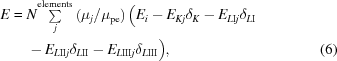where δ is the probability of K-shell (or L-shell) ionization (A) × K-shell (or L-shell) fluorescence yield (B) × fluorescent X-ray escape probability (C), and EKj (ELj) is the K-edge (L-edge) energy of element j. In general, we find that the contributions to the total fluorescence escape from the LI and LII shells are low, with LIII having higher fluorescence escape even at the LI absorption edge.

The steps employed to make this calculation in RADDOSE and the probabilities A, B, C and F are shown diagrammatically in Fig. 5. The correction factor δ may be understood as the term μabs in equation (2).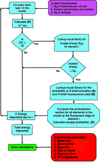Figure 5 Flow chart showing the stages used by RADDOSE to calculate the dose absorbed by a crystal. The steps involved in the fluorescence escape corrections are coloured blue. Initially the contribution of a given element to the absorption coefficient is computed (F). The unit-cell size and contents of the crystal influence this term. If the absorption edge for an element can be reached by the incident beam energy, the relevant photoelectric cross sections [obtained from the mucal library (McMaster et al., 1969)] can be used along with the probabilities of ionization, fluorescence yield, crystal thickness and absorption coefficient at the fluorescence energy to calculate the probability of fluorescent escape for this element.

### 4. Results and discussion

The aim of the program RADDOSE is to obtain an accurate estimate of the absorbed dose. To quantify and illustrate the effect of X-ray fluorescence escape on the dose, six complementary examples (Table 2) of macromolecular crystals containing heavy-atom or anomalous scatterers were subject to computational treatment with the old and new versions of RADDOSE. A default crystal size of 0.1 × 0.1 × 0.1 mm was assumed for all examples (unless otherwise stated), with a uniform (top-hat) beam profile and a flux of 1012 photons s−1 though 100 × 100 µm slits (representing typical conditions on beamline ID14-4 at the ESRF). An accurate estimate of the beam flux may be obtained from a beamline counter reading calibrated against a reference photodiode at the crystal position (Owen et al., 2009).

 Table 2Summary of examples used to illustrate the effect of fluorescence escape on absorbed dose calculations
 All calculations were performed with a crystal size of 0.1 × 0.1 × 0.1 mm and a beam size of 100 × 100 µm with a top-hat profile unless stated otherwise in the text.
Dominant atom undergoing X-ray fluorescence escape Estimated factor by which lifetime increases when
Protein Energy (keV) Element Edge type (and edge energy) (keV) X-ray fluorescence escape is included
Ferritin 12.4 Fe K (7.11) 1.14
PPK 12.6634 Se K (12.6) 1.37
Dsk2 UBA 12.66 Se K (12.6) 1.22
HPBP 17.17 U LIII (17.16) 1.21
Urate Oxidase 7.24 Gd LIII (7.24) 1.03
TMPy 13.49 Br K (13.4) 1.32

#### 4.1. The iron storage protein ferritin

Ferritin is an iron storage protein of monomeric mass 19.8 kDa, forming a 24-mer spherical conformation of internal diameter 78 Å, and contains a variable number of iron (III) atoms, from 0 in the case of apoferritin to ∼2000 for holoferritin (Owen et al., 2006). This extremely high heavy-atom content (one Fe atom per two amino acid residues) makes holoferritin an ideal system to illustrate quantitatively the effect of X-ray fluorescence in absorbed dose calculations. There is no effect of X-ray fluorescence below the Fe edge (7.11 keV) (Fig. 6). Upon reaching the K-edge of Fe, about 20% of the absorbed energy escapes as X-ray fluorescence. Away from the fluorescence edge the contribution of X-ray fluorescence escape decreases gradually from 18% (8 keV) to 10% (15 keV).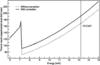Figure 6 The effect of taking into account X-ray fluorescence escape on the time taken to reach 30 MGy, the experimental dose limit, can clearly be seen above the iron K-edge (7.11 keV) for holoferritin. The new treatment used by RADDOSE shows that the absorbed dose was previously overestimated by 13% (at 13.2 keV) if fluorescence was not taken into account.

Although holoferritin is an extreme example of high metal content in a protein, the above calculations show that only a small fraction of the atoms undergo X-ray fluorescence at the energies used for a typical MX data collection. Thus, the energy lost owing to fluorescence escape is minimal for typical macromolecular crystals since they usually contain just a few metal ions in their unit cell in contrast to holoferritin. It should be noted that the effects of X-ray fluorescence escape of the Fe and Cd atoms were included via manual calculation in the absorbed dose calculations for the holoferritin crystals presented by Owen et al. (2006). At 12.4 keV this manual correction, carried out as described in detail in §3, resulted in a reduction of the calculated absorbed dose for holoferritin crystals by approximately 14%.

#### 4.2. Selenomethionine phosphomethylpyrimidine kinase

As shown above, the fluorescence correction makes the largest difference to the calculated dose at energies at and above the K-edge of the heavy element in the crystal. A common observation is that selenomethionine (SeMet) crystals have a shorter lifetime in the beam than the corresponding native crystal, owing to the large photoelectric cross section of selenium. To illustrate this, the crystal composition of a putative phosphomethylpyrimidine kinase protein (PPK) from Thermotoga maritime (Rudiňo-Piňera, unpublished results) was used. Crystals of size 0.04 × 0.1 × 0.05 mm grew in the triclinic space group P1 with four monomers in the unit cell each containing 398 residues (mass 45 kDa) with three cysteines and 12 methionines (excluding the N-terminal methionine residue). The SeMet crystal contained five S and ten Se atoms per monomer as revealed by a microPIXE measurement (Garman & Grime, 2005).

As expected, absorbed dose calculations for native and SeMet PPK crystals revealed a large increase in absorbed dose for SeMet PPK, leading to a significant decrease in the time taken to reach the experimental dose limit (Fig. 7) for the derivative. Table 3lists the relative contributions of the component atoms when the data are collected for the native and SeMet derivatised crystal at different energies, and with and without the fluorescent escape correction. Above the K-edge energy of Se, a large contribution to the absorbed dose is due to Se atoms in the crystal.

 Table 3Calculations including and excluding fluorescence escape for native and selenomethionine derivatised crystals of PPK at energies around the selenium edge
 Native crystals with and without fluorescence escape estimation show similar absorption due to the fact that there is only a tiny probability of fluorescence escape for light atoms.
Average energy deposited per photon Contribution of the various atoms to the absorbed energy (%) Absorbed dose (MGy) Time taken (s) to reach
Crystal Fluorescence correction Energy (keV) absorption event (keV) Se S P O N C per 1 s exposure experimental limit (30 MGy)
SeMet Yes 12.6 12.6 10 1 2 70 6 12 0.16 187
SeMet Yes 12.6609 10.1 33 1 1 51 5 9 0.189 158
SeMet No 12.6609 12.66 40 1 1 46 4 8 0.237 126
SeMet Yes 12.6634 9.2 46 1 1 41 4 7 0.223 134
SeMet No 12.6634 12.66 54 1 1 36 3 6 0.306 98
Native Yes 12.6634 12.66 1 2 77 7 13 0.144 207
Native No 12.6634 12.66 1 2 77 7 13 0.144 207
SeMet Yes 12.72 10.0 35 1 1 50 5 8 0.193 155
SeMet No 12.72 12.72 42 1 1 44 4 7 0.245 121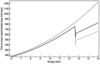Figure 7 The effects of X-ray fluorescence on crystal lifetime can be clearly seen above for native (grey) and SeMet (black) protein PPK. Unbroken and dotted lines indicate calculations including and excluding the phenomenon of X-ray fluorescence escape for the SeMet protein, respectively. Owing to the absence of heavy atoms, there are no differences in the calculated doses for the native crystal.

This example shows that inclusion of the fluorescence escape in RADDOSE calculations is important for predicting the maximum crystal lifetime for a Se-MAD experiment where data at several different wavelengths are required, since the predicted maximum lifetime increases by 27% at 12.6634 keV when the escape is included in the calculation compared with when it is not.

#### 4.3. C-terminal of UB-associated domain from S. cerevisiae

Another example of a SeMet phase determination was that of the crystal structure of Dsk2 UBA (ubiquitin-associated domain) [Protein Data Bank (PDB) code 2bwb] (Lowe et al., 2006) that had eight selenium sites. This domain consists of approximately 45 residues and crystallizes in the monoclinic space group C2 with nine molecules in the asymmetric unit. Comparison of dose calculations including and excluding fluorescence escape at the energies around the selenium edge shows that not taking fluorescence escape into account leads to an overestimate in the absorbed dose by approximately 17% (Table 4). The expected lifetime thus increases by 17% for both peak and high-energy remote collections.

 Table 4Estimated absorbed dose for SeMet* and nativeN Dsk2 UBA protein
 + and − signs indicate dose calculations including and excluding the effects of X-ray fluorescence escape, respectively.
Absorbed dose (105 Gy) per 1 s exposure Time taken to reach experimental dose limit of 30 MGy (s)
Energy (keV) + +
12.66* (peak) 0.54 0.45 552 673
13.66* (high-energy remote) 0.47 0.39 638 772
13.27N (native) 0.32 0.32 941 941

#### 4.4. Human phosphate-binding protein

To highlight the importance of including X-ray fluorescence escape for data collections performed at the LIII-edge, the effect on the lifetime of crystals of human phosphate-binding protein (HPBP) was investigated. The structure of HPBP was solved to 1.8 Å using the SIRAS (single isomorphous replacement anomalous scattering) method from uranyl heavy-atom derivatives (Morales et al., 2006). Small crystals of 0.05 × 0.05 × 0.05 mm were used to collect data at three different wavelengths (FIP BM30, ESRF). Table 5shows that 17.7% of the absorbed energy may escape the crystal at the LIII absorption peak (17.17 keV) in the form of X-ray fluorescence. There is no K-shell X-ray fluorescence from uranium at energies routinely used in MX (5–18 keV). In the absence of the experimental fluorescence scan data from the actual data collection at 17.15 keV, which is just below the LIII absorption edge of uranium at 17.17 keV (obtained from the edge energy database), a data collection at the LIII peak energy of 17.17 keV has been simulated to quantify the effects of X-ray fluorescence on the absorbed dose. From the RADDOSE calculations a 53% increase in absorbed dose is predicted between native and uranyl derivative crystals at the LIII-edge when the fluorescent escape is included whereas this increase is 62% if fluorescence escape is not considered.

 Table 5Effect of the fluorescent escape correction on the predicted maximum lifetime for a uranium-containing sample when collecting data close to the LIII-edge from HPBP crystals, keeping all other parameters constant
 All uranium contributions have been calculated from doses which take account of fluorescence escape. Although a smaller crystal was specified in these calculations, the same beam parameters (100 × 100 µm, 1012 photons s−1) were used as in the other examples.
Absorbed dose (105 Gy) per 1 s exposure Time taken to reach experimental dose limit of 30 MGy (s) Energy lost through LIII X-ray Contribution of uranium to total
Energy (keV) and crystal type + + fluorescence escape (%) dose absorbed by crystal (%)
13.27 (native) 0.296 0.296 1013 1013
17.15 (uranyl) 0.283 0.283 1058 1058 0 42
17.17 (uranyl, peak) 0.435 0.358 689 837 17.7 59
17.25 (uranyl, remote) 0.432 0.355 694 843 17.7 59

Fig. 8shows the absorbed dose of the native and derivative crystals over the incident X-ray energy range 8–25 keV. The absorbed dose shows an increase of 21, 19 and 10% at the LIII, LII and LI absorption edge peaks, respectively, owing to the increase in the photoelectric cross section of uranium.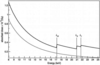Figure 8 Graph showing the absorbed dose for native (grey) and uranyl derivative (black) crystals of HPBP for the experimental conditions described in the text.

The high anomalous signal from heavy-atom derivatives with accessible L-edges provides an excellent tool for phasing, especially when used in conjunction with RIP (Fütterer et al., 2008). Gadolinium has an intense white line at the LIII-edge at 7.24 keV, which could result in a very strong signal for phasing. At this incident energy the effect of X-ray fluorescence escape on a 0.1 × 0.1 × 0.1 mm 100 mM gadolinium soaked urate oxidase (Girard et al., 2003; Murray et al., 2004) crystal is only a 3% decrease, even though the Gd atoms contribute up to 25% of the absorbed dose. This surprisingly small change can be attributed to the large photoelectric cross section of Gd (103168 barns atom−1 at 7.24 keV) leading to a very high μabs (1.61 mm−1). Most of the fluorescent X-rays are thus absorbed in the crystal. For U, the photoelectric cross section at 17.17 keV (its LIII-edge) is much lower (38426 barns atom−1) (1 barn = 10−28 cm2 and μabs = 0.19 mm−1 for this example).

#### 4.5. Nucleic acid crystals

Performing halide soaks into nucleic acid crystals is a convenient method of experimental phasing to determine the structures of nucleic acids. The crystal structure of a B-type DNA hexanucleotide duplex (PDB code 1em0) complexed with the porphyrin molecule nickel-(tetra-N-methylpyridyl) porphyrin (TMPy) was solved to 0.86 Å (Bennett et al., 2000). Data were collected at four different wavelengths (13.48, 13.49 and 16 keV on BM14, ESRF, and 13.69 keV on X11, DESY) chosen from a crystal fluorescence spectrum and the phases determined by treating the MAD data as multiple isomorphous replacement data. The asymmetric unit of the crystal contained two duplexes of the self-complementary hexamer 5-d(BrCCTAGG) with four bromine, two nickel and one magnesium atom each. Assuming a 0.1 × 0.1 × 0.1 mm crystal, the absorbed dose values with and without consideration of fluorescent escape are given in Table 6. RADDOSE calculations at these incident X-ray energies reveal that 20–24% of the absorbed energy escapes from the crystal via X-ray fluorescence. At the remote energy (16 keV), there is a 22% increase in time taken to reach the experimental dose limit, whereas at energies close to the Br K-edge (13.4 keV) a 24% increase is predicted.

 Table 6Absorbed dose calculations for TMPy crystals at three different wavelengths including (+) and excluding (−) the effect of fluorescence escape
 The high-resolution data (0.86 Å) used in refinement of the crystal structure were collected at 0.9057 Å (13.69 keV).
Absorbed dose (106 Gy) per 1 s exposure Time taken to reach experimental dose limit of 30 MGy (s)
Energy (keV) + +
13.48 (maximum) 0.13 0.095 238 314
13.49 (mid point) 0.13 0.095 238 315
13.69 0.12 0.093 244 321
16.0 (remote) 0.094 0.074 318 406

### 5. Discussion

The above results have illustrated that inclusion of the fluorescent X-ray escape in RADDOSE has the effect of significantly reducing the calculated absorbed dose for crystals containing heavier elements. The magnitude of the reduction depends on both the crystal thickness and the energy difference between the incident X-ray and the absorption edge of that element. It is clear that, for accurate dose calculations for heavy-atom-containing crystals, this effect is a necessary component of the RADDOSE algorithm, despite the fact that in all cases exemplified above the majority of the energy deposited by the beam remains in the crystal and is not lost due to X-ray fluorescence escape.

The high photon fluxes available from third-generation synchrotrons have been used successfully for de novo phasing especially using MAD and SAD techniques. These methods rely on obtaining a strong signal when data are collected at the absorption peak of the heavy element. Heavier atoms have large photoelectric cross sections which are larger than all the light atoms in a macromolecule combined. Particularly strong absorption is observed for elements heavier than tantalum (Z = 73) when data are collected at the LIII-edge. Although the dose is reduced by the fluorescence escape of some X-rays, the presence of these heavier atoms always results in greater absorption of the beam (e.g. Fig. 8), with concomitant shorter predicted lifetimes than for native crystals of the same protein, especially on or above the absorption peak of the heavy element.

The extra absorption caused by any non-specifically bound heavier atoms can be reduced by back-soaking. This technique is strongly recommended where appropriate, since these heavy atoms contribute to the absorbed dose and diffuse background scatter but not to the diffraction signal.

It should also be noted that, since the probability of fluorescent escape is greater for thinner crystals, there is a larger reduction in absorbed dose for such crystals, thus extending their predicted lifetime by more than for a thicker crystal.

Some further development of the RADDOSE program is still necessary to improve dose estimates for MX experiments. An assumption currently made is that the crystal remains stationary during the irradiation. For a crystal that is smaller than the beam, this gives no error. However, when the crystal is bigger than the beam size, this supposition causes the dose to be overestimated since, as the crystal is rotated, new un-irradiated parts of it enter the beam. In order to take this effect into account, both the dimensions of the crystal and the orientation of these dimensions relative to the rotation axis must be known. Beamlines currently do not have the facility to easily obtain this information, but such tools are being developed. Once they are available, a proper calculation of the irradiated volume within RADDOSE and thus of the correct dose will be possible. With the advent of more microfocus beamlines, where the beam will nearly always be smaller than the crystal, it will be important to incorporate this feature into RADDOSE to allow realistic estimates of the dose and the maximum crystal lifetime. It will also allow better values to be obtained for conventional beamlines where plate-like or needle crystals bigger than the beam are utilized.

An error analysis of the dose estimates produced by RADDOSE has been performed and, apart from the swept volume error described above, the uncertainty is dominated by the photon flux calibration. A 10% variation in this feeds through directly to a 10% change in the absorbed dose. Thus the importance of proper beamline flux calibration in relation to accurate dose calculations cannot be overestimated, and simple methods have now been validated for such measurements (Owen et al., 2009).

### 6. Conclusions

The potential to carry out quantitative and qualitative assessment of radiation damage as a function of dose is of increasing importance owing to the widespread use of synchrotron radiation for MX and the recognition that radiation damage can give artefacts in biological structures. At incident energies close to the absorption edges of elements present in the crystal, it is especially useful to know the relative predicted doses that will be absorbed by the crystal at different energies. This can inform the optimum data acquisition strategy and the order in which the data sets are collected.

RADDOSE calculations provide reasonable estimates for the dose absorbed by a crystal, given the caveats above regarding the swept volume problem and the fact that the energy loss in the crystal owing to the Compton effect is neglected. Thus a maximum tolerable dose for a given crystal can be calculated, after which the biological information might be compromised.

The results of the fluorescent escape treatment presented here reveal that some of the fluorescent X-rays can escape from the crystal, leading to lower absorbed doses than previously thought. In particular, for SeMet proteins there is a non-negligible effect owing to X-ray fluorescence escape that results in increased maximum lifetime predictions for such crystals compared with previous calculations. The analysis above also illustrates that fluorescence energy losses are more from crystals of smaller dimensions than larger ones and for crystals containing heavier elements.

It should be emphasized that dose calculations such as those performed by RADDOSE only take into account the physics of the energy loss of the beam in the crystal, and do not include any consideration of the chemistry or environment of the macromolecule, e.g. the possibility that particularly susceptible residues are at crystal contacts which, when damaged, will result in the disintegration of the lattice prior to reaching the dose limit. Radiation damage may become apparent at significantly lower doses since active and/or metal sites are particularly susceptible to specific X-ray-induced changes, and may be modified well before the dose limit is reached. The quantities calculated by RADDOSE are not able to provide the experimenter with any indication of the dose at which these changes occur.

The new version of RADDOSE, which is now independent of CCP4 input routines, is freely available and can be obtained from elspeth.garman@bioch.ox.ac.uk.

### Footnotes

Present address: Diamond Light Source Ltd, Diamond House, Harwell Science and Innovation Campus, Didcot, Oxfordshire OX11 0DE, UK.

### Acknowledgements

We thank Raimond Ravelli and Ian Carmichael for constructive discussions on the fluorescence escape parameterization, and Ian Carmichael for helpful comments on the manuscript. Ed Lowe and Enrique Rudiňo-Piňera are thanked for providing information for the examples. KSP is supported by the EU Sixth Framework Programme TotalCryst.

### ReferencesJOURNAL OFSYNCHROTRONRADIATION